Courses

# Balancing of Redox Equations - Redox Reactions, CBSE, Class 11, Chemistry | EduRev Notes

## Class 11 : Balancing of Redox Equations - Redox Reactions, CBSE, Class 11, Chemistry | EduRev Notes

The document Balancing of Redox Equations - Redox Reactions, CBSE, Class 11, Chemistry | EduRev Notes is a part of the Class 11 Course Chemistry for JEE.
All you need of Class 11 at this link: Class 11

Balancing of Redox Chemical Equations

Every chemical equation must be balanced according to law of conservation of mass. In a balanced chemical equation, the atoms of various species involved in the reactants and products must be equal in number. Redox reaction can be balanced through (i) Ion electron method (ii) Oxidation number method

Ion-Electron Method:

This method of balancing was developed by Jette and Lamer in 1927.

For example: balance the equation

Cu + HNO3 → Cu(NO3)2 + NO + H2O

It involves the following steps:

Step I: Write the redox reaction in ionic form.

Cu + H+ + NO3 → Cu2+ + NO + H2O

Step II: Split the redox reaction into its oxidation-half and reduction half-reaction.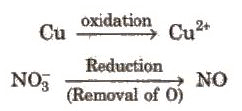Step III: Balance atoms of each half-reaction (except H and O) by using simple multiples.

Cu → Cu2+ and NO3 → NO

(Except H and O, all atoms are balanced)

Step IV: Balance H and O as

(i) For acidic and neutral solutions- Add H2O molecule to the side deficient in oxygen and H+ to the side deficient in hydrogen.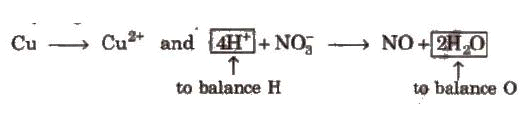(ii) For alkaline solutions- For each excess of oxygen, add one water molecule to the same side and OH ion to the other side to balance H.

Step V: Add electrons to the side deficient in electrons.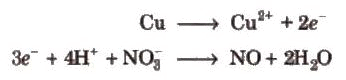Step VI: Equalize the number of electrons in both the reactions by multiplying a suitable number.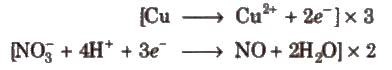Step VII: Add the two balanced half-reactions and cancel common terms of opposite sides.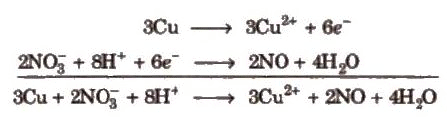Step VIII: Convert the ionic reaction into molecular form by adding spectator ions.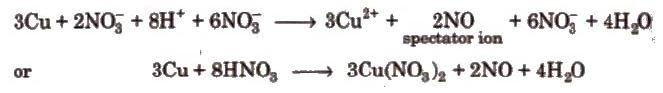(Ions which are present in solution but do not take part in the redox reaction are omitted while writing the net ionic equation of a reaction and are known as spectator ions.)

Oxidation Number Method:

For example, balance the equation,

Mg + HNO3 → Mg(NO3)2 + N2O + H2O

It involves the following steps.

Step I: Write the skeleton equation (if not given).

Step II: Assign oxidation number of each atom.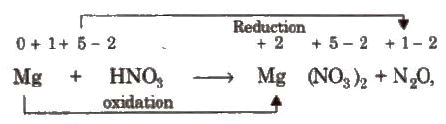Step III: Balance atoms other than H and O in two processes.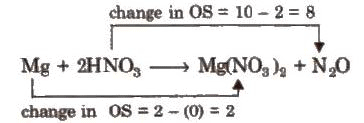Step IV: Equalize the total increase or decrease in oxidation number.

4Mg + 2HNO3 → 4Mg(NO3)2 + NO2O

Step V: Balance H and O

8H+ + 4 Mg + 2HNO3 + 8NO3 → 4 Mg (NO3)2 + N2O + 5H2O

4 Mg + 10 HNO3 → 4 Mg (NO3)2 + N2O + 5H2O

Redox Reactions in Daily Life: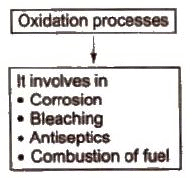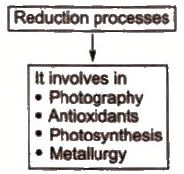Offer running on EduRev: Apply code STAYHOME200 to get INR 200 off on our premium plan EduRev Infinity!

## Chemistry for JEE

223 videos|452 docs|334 tests

,

,

,

,

,

,

,

,

,

,

,

,

,

,

,

,

,

,

,

,

,

,

,

,

,

,

,

,

,

,

;